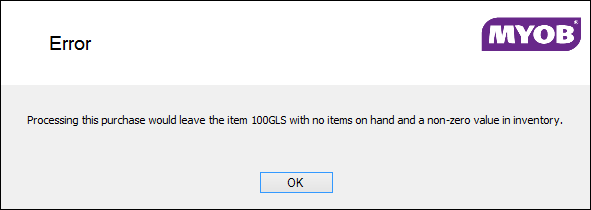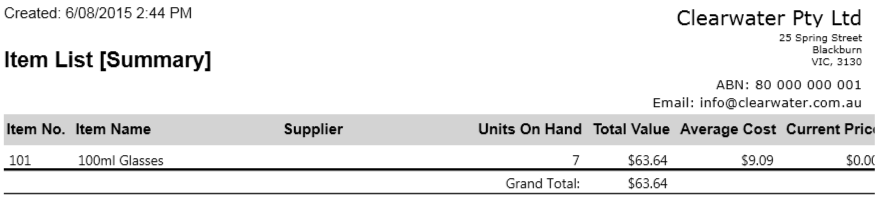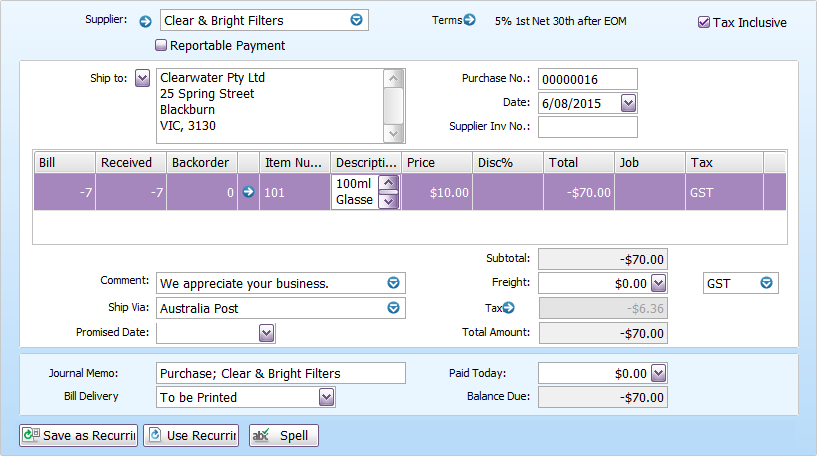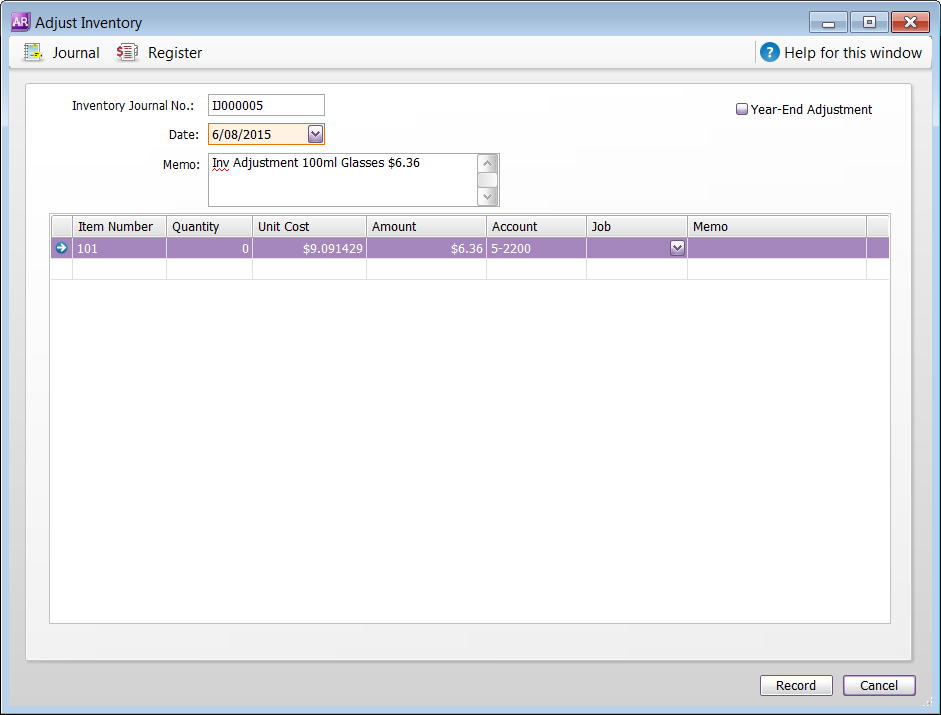##### Page tree

You are viewing an old version of this page. View the current version.

Version 46

Close

Just these help pages, not phone support or the product itself

0
1
2
3
4
5
Very dissatisfied
Very satisfied

Why did you give this rating?

Anything else you want to tell us about the help?

If you try to record a transaction that would result in a dollar value with no units on hand, you will receive the following error.This means that while your inventory quantity is zero, you still have a positive dollar value attached to the item.

Managing a full inventory can sometimes be tricky. The MYOB Inventory Training Course is an excellent resource to better understand AccountRight's inventory and its features - helping you stock smarter.

### Why would the average cost be different than the return price?

AccountRight uses the average costing method to track inventory values, which, unlike the first in first out (FIFO) method, calculates the cost of each item in your inventory by using the average cost of the whole group of items in stock.

As the price of the stock you buy changes over time, the average cost of your stock will also change. Problems can occur when your average price doesn't match the actual price of a transaction you're recording. For example, you need to return stock at the original price but it's different to the current average cost. See below for more examples.

Understanding average cost

AccountRight uses the average costing method of inventory, which values your individual inventory based on the average unit price. The formula for calculating an item's average cost is:

Average Cost = Total Value of the item divided by the Total Quantity of the items

For example, a purchase of 10 items for \$10.00 (tax exclusive) will result in an average cost of \$10.00 per item. If the following day another 10 items were purchased for \$12.00 (tax exclusive), then:

• The total quantity of the items on hand = 20 items.

• The total value of the items = \$ 220.00.

• The average cost of the items = \$11.00 (\$220.00/20)

Complications can sometimes occur if differently priced items are taken out of the inventory and others are returned, while the item's average cost would remain the same.

In the example above, say after the second purchase, 10 items were sold, the 10 remaining items would retain their average cost of \$11.00 (\$110.00 Total Value). If the remaining 10 items were to be returned to the supplier at a price of \$12.00 each (\$120.00 Total Value), then the debit purchase note will result in a:

• zero quantity, and
• a less than zero dollar value.

Because of the less than zero value, the negative inventory error will be displayed. To correct this, an inventory adjustment will first be needed to increase the Total Value of the items. The inventory adjustment will show zero quantity, a zero unit cost and a positive \$10.00 in the amount column.

### How can I fix it?

Before you can record this transaction, you need to adjust your inventory value by the amount that it's unbalanced by. Follow the steps below to find the adjustment amount and record the inventory adjustment.

1. Run an inventory report

Run the Item List [Summary] report for the applicable items.

1. Go to the Reports menu and choose Index to Reports.
2. Click the Inventory tab.
3. Click the Item List [Summary] report (under the Items sub-heading) then click Advanced.
4. At the Items field, select the items you want included in the report.
5. Select the option Include Zero Quantities.
6. Click Run Report.
2. Compare the report with your transactions

You'll now be able to compare the value of the items on the Items List [Summary] with those of the transaction. The quantity should be the same, but the dollar value will be greater or less.

Compare the value of the items from the Items List [Summary] report, with those of the transaction. You will see that the quantity will be the same (zero), but the dollar value will be greater or less.

For instance, Clearwater Pty Ltd want to return the remainder of their 100ml glasses to their supplier. 7 glasses need to be returned at their original cost of \$10.00. When they try to record the return, a non-zero value alert is given.

The example below shows the Items List [Summary] report filtered for the glasses.The list shows that the average cost of the glasses are \$9.09. If the return was recorded, the total value of the glasses would drop below zero (-\$6.36), even though the quantity would be 0.This comparison shows that the total value needs to be increased by \$6.36, so an inventory adjustment needs to be done.

An inventory adjustment is used to increase the dollar value of the items. Keep the quantity of the items at 0, because we don't want to add any more into the inventory.

Next, you'd increase the amount by the difference you found in the previous task. In our example, it was \$6.36.

Finally, you'd enter the account you want to assign the adjustment amount to. This account is usually a cost of sales or inventory adjustment account.From the community

 Negative inventory Value Strange Error in Stock On Hand after Stock take - a Negative Figure Negative inventory on-hand values block upgrading from v19.16 to 2021.1 Analyse Inventory report showing negative stock orders inventory accrual issues